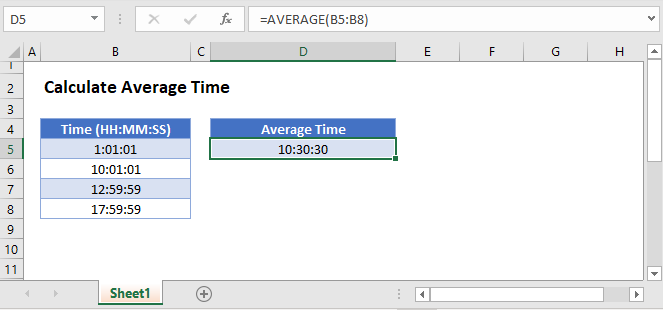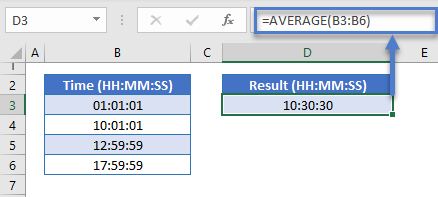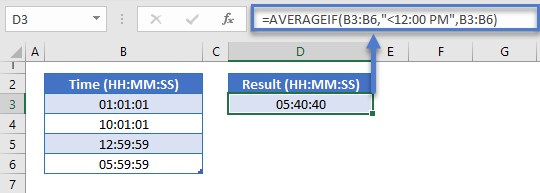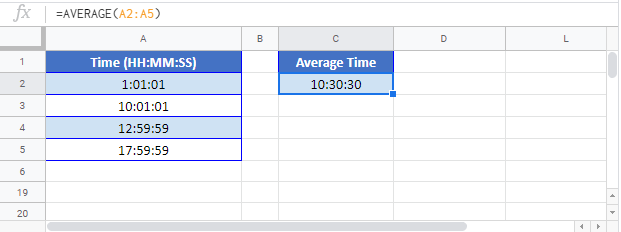# Calculate Average Time – Excel & Google Sheets

This tutorial will demonstrate how to calculate the average time in Excel & Google Sheets.To calculate the average time in Excel, simply use the Average Function over a range of times:

``=AVERAGE(B3:B6)``### Average Time With Condition

You can also use the AVERAGEIF Function to average a range of times that meet certain criteria.  In this case let’s average only times in the morning (before 12:00pm):

``=AVERAGEIF(B3:B6,"<12:00 PM",B3:B6)``## Averaging Time in Google Sheets

All of the above examples work exactly the same in Google Sheets as in Excel.### Excel Practice Worksheet

Practice Excel functions and formulas with our 100% free practice worksheets!